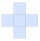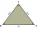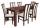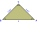# Perimeter + expression of a variable from the formula - math problems

#### Number of examples found: 110

• Triangle perimeterCalculate the triangle perimeter whose sides are in ratio 3: 5: 7 and the longest side is 17.5 cm long.
• CircleHow big is area of circle if its circumference is 80.6 cm?
• Cross fiveThe figure on the picture is composed of the same squares and has a content of 45cm². What's its perimeter?
• Cube 7Calculate the volume of a cube, whose sum of the lengths of all edges is 276 cm.
• Equilateral triangleCalculate the area of an equilateral triangle with circumference 72cm.
• TrianglesEquilateral triangle with side 40 cm has the same perimeter as an isosceles triangle with arm of 45 cm. Calculate the base x of an isosceles triangle.
• Sides of triangleTriangle circumference with two identical sides is 117cm. The third side measures 44cm. How many cms do you measure one of the same sides?
• Diamond and diagonalsA diamond has diagonals f = 8 cm and g = 6 cm long. How long is this diamond perimeter? (Calculate it!)
• TableThe circumference of the rectangle table is 420 cm. Length to width ratio is 5:2 . Calculate table dimensions and dimensions reduced in the ratio 3:5
• The perimeter 3The perimeter of a rectangle is 35 cm. The ratio of the length to its width is 3:2. Calculate the dimensions of the rectangle
• Square s3Calculate the diagonal of the square, where its area is 0.49 cm square. And also calculate its circumference.
• Sum of the edgesThe sum of the lengths of all edges of the cube is 72 cm. How many cm2 of colored paper are we going to use for sticking?
• Isosceles triangleIn an isosceles triangle, the length of the arm and the length of the base are in ration 3 to 5. What is the length of the arm?
• Perimeter of RTFind the circumference of the rectangular triangle if the sum of its legs is 22.5 cm and its area is 62.5 cm2.
• Find theFind the radius of the circular base of the vessel, whose perimeter is 2.51 m. Write the result to one decimal place in meters. Via the π sign - Ludolph's number
• PerimetersA rectangle has a perimeter of 16p centimeters, it had a width of 2p centimeters. Each side of an equilateral triangle is 1/2 the length of the rectangle. Find the total perimeter of the rectangle and the triangle if p=8.
• Diagonals of diamondFind the area and circumference of the diamond ABCD with 15m and 11m diagonals.
• FloorRectangular floor of living room has a length 5.4 meters and a circumference 17.2 meters. What is its width?
• RT 11Calculate the area of right tirangle if its perimeter is p = 45 m and one cathethus is 20 m long.
• In aIn a triangle, the aspect ratio a: c is 3: 2 and a: b is 5: 4. The perimeter of the triangle is 74cm. Calculate the lengths of the individual sides.

Do you have an interesting mathematical word problem that you can't solve it? Submit a math problem, and we can try to solve it.

We will send a solution to your e-mail address. Solved examples are also published here. Please enter the e-mail correctly and check whether you don't have a full mailbox.

Please do not submit problems from current active competitions such as Mathematical Olympiad, correspondence seminars etc...